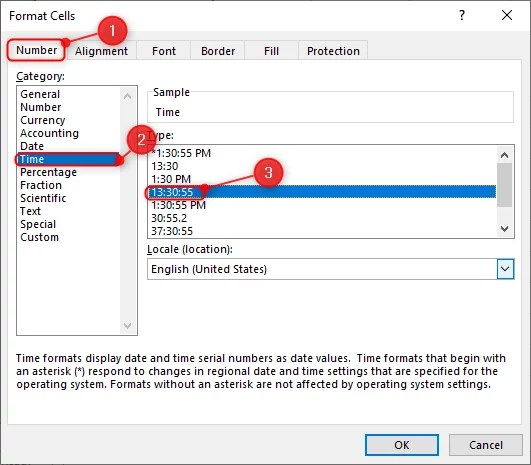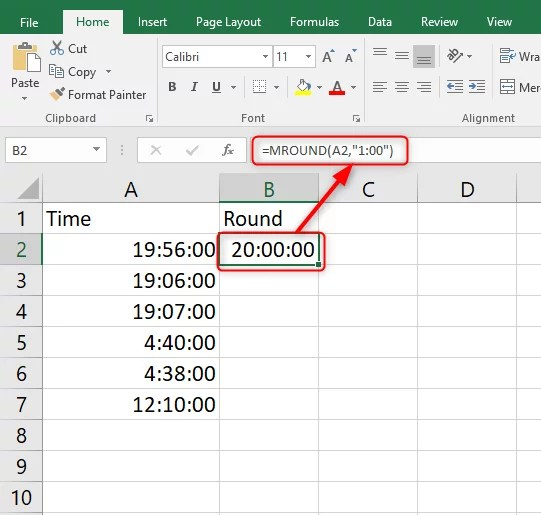# How to round time in Excel to the nearest hour (7 Formulas)

Sometimes when working with Microsoft Excel, there may be situations where you may need to round time values. Luckily, Excel offers different rounding functions you can use depending on your purpose.

In our guide below, we give step-by-step methods you can use to round time in Excel to the nearest hour. Here we go.

## Using the MROUND function

Start by formatting your cells as time formats before using the MROUND function.

1. Select all the cells that you need the values rounded to the nearest hour.

2. Right-click on the selection to display a context menu list.

3. Click on the Format Cells option to display a dialog window.

4. In the Format Cells pop up, click on the Number

5. Under the Category section, click on

6. Select a time type section7. Click the OK

Applying the formula

1. In an open Excel worksheet, click on an empty cell where you want your results displayed.

2. Type in your MROUND formula starting with the equal sign. Here is how it will be like =MROUND(

3. Next, reference the cell you want, rounding off to the nearest hour.

4. After the comma, write your condition in double quotes. In our case, it will be 1 minute which will be “1.00”.

5. Close the bracket and press the Enter key.6. To apply this formula to the other cells, use the Fill Handle.

## Using the ROUND function

You can use the Round formula to round off time up or down the nearest hour. Let’s say you have your time values in cell A3 that you need to round to the nearest hour. Using the ROUND function, here is how your formula will be:

`=ROUND(A3*24,0)/24`Here, we times time by 24 hours.  This helps to change a serial number to hours. We divide our value by 24 hours to convert our results value back to the time format.

## Rounding time down to the nearest hour

To round time down to the nearest hour, we use the ROUNDDOWN and FLOOR functions. Using our example above, here is what our formulas will look like:

=ROUNDDOWN (A3*24, 0)/24

=FLOOR (A3, “1:00”)

=FLOOR (A3, TIME (1, 0, 0))

=FLOOR (A3, 1/24)

## Rounding time up to the nearest hour

When rounding time up to the nearest hour, we use the CEILING and ROUNDUP functions. Here is an example of what your formulas should look like:

=ROUNDUP (A3*24, 0)/24

=CEILING (A3,” 1:00″)

=CEILING (A3, TIME (1, 0, 0))

## Using the TIME formula

You can use Excel’s TIME formula to round time . These functions include the TIME, MINUTE, HOUR, and SECOND functions.

For instance, using the above example of cell B3, here is what your formula will be like:

`=TIME (HOUR (B3) + ((MINUTE (B3) +SECOND (B3)/60)>30), 0, 0)`